• 2019-03-19 09:14:07

@R语言相关分析与典型相关分析

#相关分析与典型相关分析
#pearson相关系数
a=c(1,3,5,7,9);b=c(1,4,6,9,10)
cor(a,b) #pearson相关系数
cor.test(a,b) #检验相关系数的显著性
cor(iris[1:4]) #相关系数,参数填数据集，则计算相关系数矩阵

#spearman相关系数,亦即秩相关系数
#spearman和kendall都是等级相关系数，亦即其值与两个相关变量的具体值无关，而仅仅与其值之间的大小关系有关。
#spearman相关系数，亦即秩相关系数，根据随机变量的等级而不是其原始值衡量相关性的一种方法。
m=c(1,2,4,3);n=c(100,101,102,103)
m1=c(30,31,35,34);n1=c(85,87,90,93)
cor(m,n);cor(m1,n1)
cor(m,n,method = “spearman”);cor(m1,n1,method = “spearman”)
cor.test(m,n,method = “spearman”);cor.test(m1,n1,method = “spearman”)
#spearman相关系数的计算可以由计算pearson系数的方法,只需要把原随机变量中的原始数据替换成其在随机变量中的等级顺序即可:

acf #自相关和协方差函数
acf(airmiles,type=‘correlation’,lag.max=10) #自相关
pacf(airmiles,lag.max=10) #偏自相关
pairs(~Sepal.Length+Sepal.Width+Petal.Length+Petal.Width,data=iris,
main=“Simple Scatterplot Matrix”) #散点图矩阵
install.packages(“scatterplot3d”) #3D散点图
library(scatterplot3d)
scatterplot3d(iris S e p a l . L e n g t h , i r i s Sepal.Length, iris Petal.Length, iris$Petal.Width) install.packages(“corrgram”) #有兴趣的同学自己练习 library(corrgram) #1、设置排序处理 corrgram(mtcars,order=TRUE) #2、设置上下三角面板形状 corrgram(mtcars,order=TRUE,lower.panel=panel.shade,upper.panel=panel.pie) #3、只显示下三角部分 corrgram(mtcars,order=TRUE,lower.panel=panel.shade,upper.panel=NULL) #4、调整面板颜色 corrgram(mtcars,order=TRUE,lower.panel=panel.shade,upper.panel=panel.pie, col.regions=colorRampPalette(c(“darkgoldenrod4”,“burlywood1”,“white”, “darkkhaki”,“darkgreen”))) install.packages(“corrplot”) library(corrplot) #1、使用不同的method绘制相关矩阵图 methods<-c(“circle”,“square”,“ellipse”,“pie”,“shade”,“color”) par(mfrow=c(2,3)) t0=mapply(function(x){corrplot(cor(mtcars), method=x,order=“AOE”)},methods) par(mfrow=c(1,1)) #2、设置method=color绘制热力矩阵图 corrplot(cor(mtcars), method=“color”, order = “AOE”,tl.col=“black”,tl.srt=45, addCoef.col=“black”,col=colorRampPalette(c("#7F0000",“red”,"#FF7F00", “yellow”,“white”, “cyan”, “#007FFF”, “blue”,"#00007F"))(20)) #3、绘制上下三角及不同色彩的相关矩阵图 library(RColorBrewer) par(mfrow=c(2,2)) corrplot(cor(mtcars),type=“lower”) corrplot(cor(mtcars),type=“lower”,order=“hclust”, col=brewer.pal(n=8,name=“RdYlBu”)) corrplot(cor(mtcars),type=“upper”,order=“AOE”, col=c(“black”,“white”),bg=“lightblue”) corrplot(cor(mtcars),type=“upper”,order=“FPC”, col=brewer.pal(n=8, name=“PuOr”)) par(mfrow=c(1,1)) d<-sqrt(1-cor(mtcars)^2) hc<-hclust(as.dist(d)) plot(hc) rect.hclust(hc,k=3) install.packages(“pvclust”) library(pvclust) cluster.bootstrap <- pvclust(mtcars, nboot=1000, method.dist=“correlation”) plot(cluster.bootstrap) pvrect(cluster.bootstrap) #自己练习部分结束 #典型相关：指两组变量之间的相关关系，不是两个变量之间相关关系，也不是两组变量之间两两组合的简单相关 #两组变量作为整体的相关性 #例如体育运动和身体状况的相关性，体育运动包括跑步，篮球，足球，乒乓球，游泳等变量，身体状况包括身高，体重，肺活量，血压等变量 #以R语言自带的iris为例 #1、提取iris的前4个数值列，并进行标准化处理 data0=scale(iris[1:4]) #2、计算这4个变量的协方差，由于经过标准化处理，这样得到的也是相关系数 M=cov(data0) #3、将M进行分块，1:2两个变量一组，3:4是另外一组，并进行两两组合 X11=M[1:2,1:2] X12=M[1:2,3:4] X21=M[3:4,1:2] X22=M[3:4,3:4] #4、按公式求解矩阵A和B A=solve(X11)%%X12%%solve(X22)%%X21 B=solve(X22)% %X21%%solve(X11)%%X12 #5、使用eigen函数求解典型相关系数如下 eV=sqrt(eigen(A)$values)
eV

#6、进行验证
#…比较A与XΛX^(-1)是否相等
round(A-eigen(A) v e c t o r s vectors%*%diag(eigen(A) values)%*%solve(eigen(A)$vectors),3) ## Sepal.Length Sepal.Width ## Sepal.Length 0 0 ## Sepal.Width 0 0 #…比较B与YΛY^(-1)是否相等 round(B-eigen(B) v e c t o r s vectors%*%diag(eigen(B) values)%*%solve(eigen(B)$vectors),3)

#…求解A对应的特征向量并计算典型向量C1
C1=data0[,1:2]%*%eigen(A)$vectors #…验证C1对应各变量的标准差是否为1，同时查看均差 apply(C1,2,sd) ##  1.041196 0.951045 apply(C1,2,mean) ##  -4.880321e-16 -2.759430e-17 #…由于均值为0，标准差不为1，这里对特征向量进行伸缩变换 eA=eigen(A)$vectors%%diag(1/apply(C1,2,sd))
#…再次验证方差和均值
C1=data0[,1:2]%
%eA
apply(C1,2,sd)

##  1 1

apply(C1,2,mean)

##  -4.667693e-16 -2.745503e-17

#…可见，特征向量已经满足要求，同理对B可得
C2=data0[,3:4]%*%eigen(B)$vectors apply(C2,2,sd) ##  0.6291236 0.2003530 apply(C2,2,mean) ##  -1.403572e-17 -9.859870e-18 eB=eigen(B)$vectors%%diag(1/apply(C2,2,sd))
C2=data0[,3:4]%
%eB
apply(C2,2,sd)

##  1 1

apply(C2,2,mean)

round(cor(cbind(C1,C2)),3)
#用cancor可以直接求解典型相关系数
x<-as.matrix(iris[,1:2])
y<-as.matrix(iris[,3:4])
cancor(x,y)

更多相关内容
• 典型相关分析 基本原理 从字面意义上理解CCA，我们可以知道，简单说来就是对不同变量之间做相关分析。较为专业的说就是，一种度量两组变量之间相关程度的多元统计方法。 关于相似性度量距离问题，在这里有一篇Blog...

随着对CCA的深入研究，是时候对CCA进行一下总结了。

本菜鸡主要研究方向为故障诊断，故会带着从应用角度进行理解。# 典型相关分析

## 基本原理

从字面意义上理解CCA，我们可以知道，简单说来就是对不同变量之间做相关分析。较为专业的说就是，一种度量两组变量之间相关程度的多元统计方法

关于相似性度量距离问题，在这里有一篇Blog可以参考参考。

首先，从基本的入手。

当我们需要对两个变量 X ， Y X，Y 进行相关关系分析时，则常常会用到相关系数来反映。学过概率统计的小伙伴应该都知道的吧。还是解释一下。

相关系数：是一种用以反映变量之间相关关系密切程度的统计指标。相关系数是按积差方法计算，同样以两变量与各自平均值的离差为基础，通过两个离差相乘来反映两变量之间相关程度；着重研究线性的单相关系数。
R ( X , Y ) = Cov ⁡ ( X , Y ) Var ⁡ [ X ] Var ⁡ [ Y ] R(X, Y)=\frac{\operatorname{Cov}(X, Y)}{\sqrt{\operatorname{Var}[X] \operatorname{Var}[Y]}}
其中， C o v ( X , Y ) Cov(X,Y) 表示 X , Y X,Y 的协方差矩阵， V a r [ X ] Var[X] X X 的方差， V a r [ Y ] Var[Y] Y Y 的方差.

复习了一下大学本科概率统计知识，那么，如果我们需要分析的对象是两组或者多组向量，又该怎么做呢？

## CCA的数学表达

这里举例两组变量 A ( a 1 , a 2 , . . . , a n ) , B ( b 1 , b 2 , . . . , b m ) A(a_1,a_2,...,a_n),B(b_1,b_2,...,b_m) ，那么我们的公式会是这样：
R ( X i , Y j ) = ∑ i = 1 , j = 1 n , m C o v ( X i , Y j ) V a r [ X i ] V a r [ Y j ] R(X_i,Y_j)=\sum_{i=1,j=1}^{n,m} \frac{Cov(X_i,Y_j)}{\sqrt{Var[X_i]Var[Y_j]}}
我们会得到一个这样的矩阵：
[ R ( X 1 , Y 1 ) . . . R ( X 1 , Y m − 1 ) R ( X 1 , Y m ) R ( X 2 , Y 1 ) . . . R ( X 2 , Y m − 1 ) R ( X 2 , Y m ) . . . . . . . . . . . . R ( X n , Y 1 ) . . . . . . R ( X n , Y m ) ] \begin{bmatrix} R(X_1,Y_1) &... & R(X_1,Y_{m-1}) & R(X_1,Y_m)\\R(X_2,Y_1) & ...& R(X_2,Y_{m-1})& R(X_2,Y_m)\\ ...& ...& ...&... \\ R(X_n,Y_1) & ...& ...&R(X_n,Y_m) \end{bmatrix}

这样的话，我们把每个变量的相关系数都求了出来，不知道会不会和我一样觉得这样很繁琐呢。如果我们能找到两组变量之间的各自的线性组合，那么我们就只分析讨论线性组合之间的相关分析。

典型相关系数：是先对原来各组变量进行主成分分析，得到新的线性关系的综合指标，再通过综合指标之间的线性相关系数来研究原各组变量间相关关系。

现在我们利用主成分分析（PCA）的思想，可以把多个变量与多个变量之间的相关转化成两个变量之间的相关。

先得到两组变量 ( A T , B T ) (A^T,B^T) 的协方差矩阵
Σ = [ Σ 11   Σ 12 Σ 21   Σ 22 ] \Sigma=\left[\begin{array}{l} \Sigma_{11} \ \Sigma_{12} \\ \Sigma_{21} \ \Sigma_{22} \end{array}\right]
其中， Σ 11 = C o v ( A ) , Σ 22 = C o v ( B ) , Σ 12 = Σ 12 T = C o v ( A , B ) \Sigma_{11} = Cov(A),\Sigma_{22} = Cov(B),\Sigma_{12}=\Sigma_{12}^T = Cov(A,B) .

把上面两组变量 A ( a 1 , a 2 , . . . , a n ) , B ( b 1 , b 2 , . . . , b m ) A(a_1,a_2,...,a_n),B(b_1,b_2,...,b_m) 分别组合成两个变量U、V，则用线性表示
U = t 1 a 1 + t 2 a 2 + . . . + t n a n , V = h 1 b 1 + h 2 b 2 + . . . + h m b m \begin{matrix} U=t_1a_1+t_2a_2+...+t_na_n,\\ \\V=h_1b_1+h_2b_2+...+h_mb_m \end{matrix}

然后，找出最大可能的相关系数 t k = ( t 1 , t 2 , . . . , t n ) T , h k = ( h 1 , h 2 , . . . , h m ) T {t_k}=(t_1,t_2,...,t_n)^T,{h_k}=(h_1,h_2,...,h_m)^T ,

使得， R ( U , V ) ⟶ M a x R(U,V)\longrightarrow Max ，这样，就得到了典型相关系数；而其中的 U , V U,V 典型相关变量

典型相关分析最朴素的思想：首先分别在每组变量中找出第一对典型变量，使其具有最大相关性，然后在每组变量中找出第二对典型变量，使其分别与本组内的第一对典型变量不相关，第二对本身具有次大的相关性。如此下去，直到进行到K步，两组变量的相关系被提取完为止，可以得到K组变量。

So

注意：此时的 ( U , V ) (U,V) 若不能反映两组变量之间的相关关系，我们需要继续构造下一组关系变量来表示，具体可构造 K K 这样的关系

直到 R ( U , V ) ⟶ M a x R(U,V)\longrightarrow Max 为止
U k = t k T A = t 1 k a 1 + t 2 k a 2 + . . . + t n k a n V k = h k T B = h 1 k b 1 + h 2 k b 2 + . . . + h m k b m \begin{matrix} U_k={t_k^T}{A}=t_{1k}a_1+t_{2k}a_2+...+t_{nk}a_n\\ \\ V_k={h_k^T}{B}=h_{1k}b_1+h_{2k}b_2+...+h_{mk}b_m \end{matrix}

其中，我们需要一个约束条件满足，使得 R ( U , V ) ⟶ M a x R(U,V)\longrightarrow Max

V a r ( U k ) = V a r ( t k T A ) = t k T Σ 11 t k = 1 V a r ( V k ) = V a r ( h k T A ) = h k T Σ 22 h k = 1 C o v （ U k , U i ） = C o v ( U k , V i ) = C o v ( V i , U k ) = C o v ( V k , V i ) = 0 ( 1 < = i < k ) \begin{matrix} Var(U_k)=Var({t_k^T}{A})={t_k^T}\Sigma_{11}t_k=1\\ \\ Var(V_k)=Var({h_k^T}{A})={h_k^T}\Sigma_{22}h_k=1\\ \\ Cov（U_k,U_i）=Cov(U_k,V_i)=Cov(V_i,U_k)=Cov(V_k,V_i)=0(1<=i<k) \end{matrix}
典型相关系数公式 R ( U , V ) R_{(U,V)}
R ( U , V ) = Cov ⁡ ( U , V ) Var ⁡ [ U ] Var ⁡ [ V ] = C o v ( U , V ) = t k T C o v ( A , B ) h k = t k T Σ 12 h k R_{(U,V)}=\frac{\operatorname{Cov}(U, V)}{\sqrt{\operatorname{Var}[U] \operatorname{Var}[V]}}=Cov(U,V)={t_k}^TCov(A,B)h_k={t_k}^T\Sigma_{12} h_k

在此约束条件下， t k , h k t_k,h_k 系数得到最大，则使得 R ( U , V ) R_{(U,V)} 最大

## 典型相关系数及变量的求法

下面一起来求 t 1 , h 1 t_1,h_1 （这里只例举第一典型相关系数）

（一起来复习高数–拉格朗日乘数法

前提条件，我们有个计算公式，约束条件也有了，故这是一个求解条件极值题呀！！！

列出我们的拉格朗日函数：
ϕ ( t 1 , h 1 ) = t 1 T Σ 12 h 1 − λ 2 ( t 1 T Σ 11 t 1 − 1 ) − v 2 ( h 1 T Σ 22 h 1 − 1 ) \phi\left(t_{1}, h_{1}\right)=t_{1}^T \Sigma_{12} h_{1}-\frac{\lambda}{2}\left(t_{1}^T \Sigma_{11} t_{1}-1\right)-\frac{v}{2}\left(h_{1}^T \Sigma_{22} h_{1}-1\right)
其中， λ , v \lambda,v 表示拉格朗日乘子参数。

由上述典型相关系数公式 R ( U , V ) R_{(U,V)} 可知，我们只需求其系数 t 1 , h 1 t_1,h_1 的最大值，即可

ϕ ( t 1 , h 1 ) \phi(t_1,h_1) 做一阶偏导运算：
{ ∂ ϕ ∂ t 1 = ∑ 12 h 1 − λ ∑ 11 t 1 = 0 ∂ ϕ ∂ h 1 = ∑ 21 t 1 − v ∑ 22 h 1 = 0 \left\{\begin{array}{l} \frac{\partial \phi}{\partial t_{1}}=\sum_{12} h_{1}-\lambda \sum_{11} t_{1}=0 \\ \\ \frac{\partial \phi}{\partial h_{1}}=\sum_{21} t_{1}-v \sum_{22} h_{1}=0 \end{array}\right.
也就是
{ ∑ 12 h 1 − λ ∑ 11 t 1 = 0 ∑ 21 t 1 − v ∑ 22 h 1 = 0 \left\{\begin{array}{l} \sum_{12} h_{1}-\lambda \sum_{11} t_{1}=0 \\ \\ \sum_{21} t_{1}-v \sum_{22} h_{1}=0 \end{array}\right.
将上式分别左乘 t 1 , h 1 t_1,h_1 得，
{   t 1 Σ 12 h 1 − λ t 1 Σ 11 t 1 = 0   h 1 Σ 21 t 1 − v h 1 Σ 22 h 1 = 0 \left\{\begin{array}{l} \ t_{1}\Sigma_{12} h_{1}-\lambda t_{1}\Sigma_{11} t_{1}=0 \\ \\ \ h_{1}\Sigma_{21} t_{1}-v h_{1} \Sigma_{22} h_{1}=0 \end{array}\right.
由于约束条件可知 V a r ( U 1 ) = V a r ( V 1 ) = 1 Var(U_1)=Var(V_1)=1 ，解得，
{   t 1 Σ 12 h 1 = λ   h 1 Σ 21 t 1 = v \left\{\begin{array}{l} \ t_{1}\Sigma_{12} h_{1}=\lambda \\ \\ \ h_{1}\Sigma_{21} t_{1} =v \end{array}\right.
此时，我们来对比一下上面列出的求解 R ( U , V ) R_{(U,V)} 公式，有没有，是不是，一模一样，我们的拉格朗日乘子 λ , v = t 1 Σ 12 h 1 = R ( U , V ) \lambda,v=t_{1}\Sigma_{12} h_{1}=R_{(U,V)} ，也就是说， λ , v \lambda,v 即为我们要求解的典型相关系数

我们由式 { ∑ 12 h 1 − λ ∑ 11 t 1 = 0 ∑ 21 t 1 − v ∑ 22 h 1 = 0 \left\{\begin{array}{l} \sum_{12} h_{1}-\lambda \sum_{11} t_{1}=0 \\ \\ \sum_{21} t_{1}-v \sum_{22} h_{1}=0 \end{array}\right. ,可得
λ t 1 = Σ 11 − 1 Σ 12 h 1 \lambda t_1 = \Sigma _{11}^{-1}\Sigma_{12} h_{1}
由于 λ = v \lambda=v ，再将此代入 ∑ 21 t 1 − v ∑ 22 h 1 = 0 \sum_{21} t_{1}-v \sum_{22} h_{1}=0 中，可得
Σ 12 Σ 22 − 1 Σ 21 t 1 − λ 2 Σ 11 t 1 = 0 Σ 11 − 1 Σ 12 Σ 22 − 1 Σ 21 t 1 − λ 2 t 1 = 0 \begin{array}{l} \Sigma_{12} \Sigma_{22}^{-1} \Sigma_{21} t_{1}-\lambda^{2} \Sigma_{11} t_{1}=0 \\ \\ \Sigma_{11}^{-1} \Sigma_{12} \Sigma_{22}^{-1} \Sigma_{21} t_{1}-\lambda^{2} t_{1}=0 \end{array}
上面的式子是不是很熟悉 A X = λ X AX=\lambda X ,

Σ 11 − 1 Σ 12 Σ 22 − 1 Σ 21 = A t 1 = X λ = λ 2 \Sigma_{11}^{-1} \Sigma_{12} \Sigma_{22}^{-1} \Sigma_{21} = A \\ t_1=X \\ \lambda=\lambda^2
故， Σ 11 − 1 Σ 12 Σ 22 − 1 Σ 21 \Sigma_{11}^{-1} \Sigma_{12} \Sigma_{22}^{-1} \Sigma_{21} 的特征值为 λ 2 \lambda^2 ，特征向量为 t 1 t_1

到此，我们可求得相应的 t 1 , h 1 t_1,h_1 R ( U , V ) R_{(U,V)}

## 典型相关分析应用

### 基于 CCA 的故障检测方法

对于CCA应用在故障检测中，基于 CCA 的故障检测方法可以视为基于 PCA 和基于 PLS 故障检测方法的一种扩展。

基本思想：是利用典型相关关系构建一个残差发生器， 通过对残差信号的评价做出故障检测的相应决策。该方法中提出了 4 个统计量， 将输入空间分为两个部分， 即与输出空间相关的子空间和与输出空间不相关的子空间；同理，将输出空间分为两个部分， 即与输入空间相关的子空间和与输入空间不相关的子空间。

u o b s ∈ Ｒ l u_{obs}∈Ｒ^l y o b s ∈ Ｒ m y_{obs}∈Ｒ^m 表示测量的过程输入和输出向量， l l m m 分别表示相应的数据维数。对两个向量进行去均值， 可得

u = u o b s − μ u (1) \boldsymbol{u} = \boldsymbol{u}_{\mathrm{obs}}-\boldsymbol{\mu}_{u} \tag{1}
y = y o b s − μ y (2) \boldsymbol{y} = \boldsymbol{y}_{\mathrm{obs}}-\boldsymbol{\mu}_{y} \tag{2}
式中: μ u μ_u μ y μ_y 分别表示输入变量均值和输出变量均值。假设采样得到 N 个过程数据， 并组成如下输入输出数据集

U = [ u ( 1 ) , u ( 2 ) , ⋯   , u ( N ) ] ∈ R l × N , Y = [ y ( 1 ) , y ( 2 ) , ⋯   , y ( N ) ] ∈ R m × N \boldsymbol{U}=[\boldsymbol{u}(1), \boldsymbol{u}(2), \cdots, \boldsymbol{u}(N)] \in \mathbf{R}^{l \times N}, \boldsymbol{Y}=[\boldsymbol{y}(1), \boldsymbol{y}(2), \cdots, \boldsymbol{y}(N)] \in \mathbf{R}^{m \times N}
式中： u ( i ) ， y ( i ) ， ( i = 1 ， … ， N ) u(i)， y(i) ， (i = 1， …， N) 是按式(1)(2)中心化后的输入输出向量， 相应的平均值
μ u ≈ 1 N ∑ i = 1 N u o b s ( i ) , μ y ≈ 1 N ∑ i = 1 N y o b s ( i ) , \boldsymbol{\mu}_{u} \approx \frac{1}{N} \sum_{i=1}^{N} \boldsymbol{u}_{\mathrm{obs}}(i), \boldsymbol{\mu}_{y} \approx \frac{1}{N} \sum_{i=1}^{N} \boldsymbol{y}_{\mathrm{obs}}(i),

并且， 协方差矩阵 Σ u 、 Σ y Σ_u、 Σ_y 和输入输出的互协方差矩阵 Σ u y Σ_{uy} 分别为:
Σ u ≈ 1 N − 1 ∑ i = 1 N ( u o b s ( i ) − μ u ) ( u o b s ( i ) − μ u ) T = U U T N − 1 (3) \boldsymbol{\Sigma}_{u} \approx \frac{1}{N-1} \sum_{i=1}^{N}\left(\boldsymbol{u}_{\mathrm{obs}}(i)-\boldsymbol{\mu}_{u}\right)\left(\boldsymbol{u}_{\mathrm{obs}}(i)-\boldsymbol{\mu}_{u}\right)^{\mathrm{T}}=\frac{\boldsymbol{U} \boldsymbol{U}^{\mathrm{T}}}{N-1}\tag{3}
Σ y ≈ 1 N − 1 ∑ i = 1 N ( y o b s ( i ) − μ y ) ( y o b s ( i ) − μ y ) T = Y Y T N − 1 (4) \boldsymbol{\Sigma}_{y} \approx \frac{1}{N-1} \sum_{i=1}^{N}\left(\boldsymbol{y}_{\mathrm{obs}}(i)-\boldsymbol{\mu}_{y}\right)\left(\boldsymbol{y}_{\mathrm{obs}}(i)-\boldsymbol{\mu}_{y}\right)^{\mathrm{T}}=\frac{\boldsymbol{Y} \boldsymbol{Y}^{\mathrm{T}}}{N-1}\tag{4}
Σ u y ≈ 1 N − 1 ∑ i = 1 N ( u o b s ( i ) − μ u ) ( y o b s ( i ) − μ y ) T = U Y T N − 1 (5) \boldsymbol{\Sigma}_{u y} \approx \frac{1}{N-1} \sum_{i=1}^{N}\left(\boldsymbol{u}_{\mathrm{obs}}(i)-\boldsymbol{\mu}_{u}\right)\left(\boldsymbol{y}_{\mathrm{obs}}(i)-\boldsymbol{\mu}_{y}\right)^{\mathrm{T}}=\frac{\boldsymbol{U} \boldsymbol{Y}^{\mathrm{T}}}{N-1}\tag{5}
结合 CCA 方法， 可得:
( U U T N − 1 ) − 1 / 2 ( U Y T N − 1 ) ( Y Y T N − 1 ) − 1 / 2 = Σ u − 1 / 2 Σ u y Σ y − 1 / 2 = Γ s Σ Ψ s T , Σ = [ Λ κ 0 0 0 ] (6) \left(\frac{\boldsymbol{U} \boldsymbol{U}^{\mathrm{T}}}{N-1}\right)^{-1 / 2}\left(\frac{\boldsymbol{U} \boldsymbol{Y}^{\mathrm{T}}}{N-1}\right)\left(\frac{\boldsymbol{Y} \boldsymbol{Y}^{\mathrm{T}}}{N-1}\right)^{-1 / 2}=\boldsymbol{\Sigma}_{u}^{-1 / 2} \boldsymbol{\Sigma}_{u y} \boldsymbol{\Sigma}_{y}^{-1 / 2}=\boldsymbol{\Gamma}_{s} \boldsymbol{\Sigma} \boldsymbol{\Psi}_{s}^{\mathrm{T}}, \boldsymbol{\Sigma}=\left[\begin{array}{ll} \boldsymbol{\Lambda}_{\kappa} & 0 \\ 0 & 0 \end{array}\right]\tag{6}
式中: κ 为主元个数， κ ≤ m i n ( l ， m ) ; Σ κ = d i a g ( ρ 1 ， … ， ρ κ ) κ ≤ min(l，m); Σ_κ= diag(ρ1， …， ρκ) 为典型相关系数值。
J s = Σ u − 1 / 2 Γ ( : , 1 : κ ) , L s = Σ y − 1 / 2 Ψ ( : , 1 : κ ) , J r e s = Σ u − 1 / 2 Γ ( : , κ + 1 : l ) , L r e s = Σ y − 1 / 2 Ψ ( : , κ + 1 : m ) \boldsymbol{J}_{s}=\boldsymbol{\Sigma}_{u}^{-1 / 2} \boldsymbol{\Gamma}(:, 1: \kappa), \boldsymbol{L}_{s}=\boldsymbol{\Sigma}_{y}^{-1 / 2} \boldsymbol{\Psi}(:, 1: \kappa), \boldsymbol{J}_{\mathrm{res}}=\boldsymbol{\Sigma}_{u}^{-1 / 2} \boldsymbol{\Gamma}(:, \kappa+1: l), \boldsymbol{L}_{\mathrm{res}}=\boldsymbol{\Sigma}_{y}^{-1 / 2} \boldsymbol{\Psi}(:, \kappa+1: m) ,

由 CCA 方法可知， J s T u J^T_su L s T y L^T_sy 具有密切的相关性。

但是在实际系统中， 测量变量难免受到噪声影响， 两者之间的相关性可表示为:
L s T y ( k ) = Λ κ T J s T u ( k ) + v s ( k ) (7) \boldsymbol{L}_{s}^{\mathrm{T}} \boldsymbol{y}(k)=\boldsymbol{\Lambda}_{\kappa}^{\mathrm{T}} \boldsymbol{J}_{s}^{\mathrm{T}} \boldsymbol{u}(k)+\boldsymbol{v}_{s}(k)\tag{7}
式中: v s v_s 为噪声项， 并且与 J s T u J^T_su 弱相关。基于此， 残差向量
r 1 ( k ) = L s T y ( k ) − Λ κ T J s T u ( k ) (8) \boldsymbol{r}_{1}(k)=\boldsymbol{L}_{s}^{\mathrm{T}} \boldsymbol{y}(k)-\boldsymbol{\Lambda}_{\kappa}^{\mathrm{T}} \boldsymbol{J}_{s}^{\mathrm{T}} \boldsymbol{u}(k)\tag{8}

其中的 Λ κ T {\Lambda}_{\kappa}^{\mathrm{T}} 为系数矩阵，上面介绍了CCA的数学运算，这里应该能理解，只不过多加了一个噪声向量。

假设输入输出数据服从高斯分布。已知线性变换不改变随机变量的分布， 所以残差信号 r 1 r_1 也服
从高斯分布， 其协方差矩阵：
Σ r 1 = 1 N − 1 ( L s T Y − Λ κ T J s T U ) ( L s T Y − Λ κ T J s T U ) T = I κ − Λ κ 2 N − 1 (9) \boldsymbol{\Sigma}_{r_1}=\frac{1}{N-1}\left(\boldsymbol{L}_{s}^{\mathrm{T}} \boldsymbol{Y}-\boldsymbol{\Lambda}_{\kappa}^{\mathrm{T}} \boldsymbol{J}_{s}^{\mathrm{T}} \boldsymbol{U}\right)\left(\boldsymbol{L}_{s}^{\mathrm{T}} \boldsymbol{Y}-\boldsymbol{\Lambda}_{\kappa}^{\mathrm{T}} \boldsymbol{J}_{s}^{\mathrm{T}} \boldsymbol{U}\right)^{\mathrm{T}}=\frac{\boldsymbol{I}_{\kappa}-\boldsymbol{\Lambda}_{\kappa}^{2}}{N-1}{ }^{}\tag{9}
同理， 还可以得到另一残差向量
r 2 ( k ) = L s T y ( k ) − Λ κ T J s T u ( k ) (10) \boldsymbol{r}_{2}(k)=\boldsymbol{L}_{s}^{\mathrm{T}} \boldsymbol{y}(k)-\boldsymbol{\Lambda}_{\kappa}^{\mathrm{T}} \boldsymbol{J}_{s}^{\mathrm{T}} \boldsymbol{u}(k)\tag{10}
其协方差矩阵
Σ r 2 = 1 N − 1 ( L s T Y − Λ κ T J s T U ) ( L s T Y − Λ κ T J s T U ) T = I κ − Λ κ 2 N − 1 (11) \boldsymbol{\Sigma}_{r_2}=\frac{1}{N-1}\left(\boldsymbol{L}_{s}^{\mathrm{T}} \boldsymbol{Y}-\boldsymbol{\Lambda}_{\kappa}^{\mathrm{T}} \boldsymbol{J}_{s}^{\mathrm{T}} \boldsymbol{U}\right)\left(\boldsymbol{L}_{s}^{\mathrm{T}} \boldsymbol{Y}-\boldsymbol{\Lambda}_{\kappa}^{\mathrm{T}} \boldsymbol{J}_{s}^{\mathrm{T}} \boldsymbol{U}\right)^{\mathrm{T}}=\frac{\boldsymbol{I}_{\kappa}-\boldsymbol{\Lambda}_{\kappa}^{2}}{N-1}{ }^{}\tag{11}
由式(9)(11) 可以看出， 残差 r1和 r2的协方差相同。 对于故障检测， 可构造如下两个统计量:
T 1 2 ( k ) = ( N − 1 ) r 1 T ( k ) ( I κ − Λ κ 2 ) − 1 r 1 ( k ) (12) T_{1}^{2}(k)=(N-1) \boldsymbol{r}_{1}^{\mathrm{T}}(k)\left(\boldsymbol{I}_{\kappa}-\boldsymbol{\Lambda}_{\kappa}^{2}\right)^{-1} \boldsymbol{r}_{1}(k)\tag{12}
T 2 2 ( k ) = ( N − 1 ) r 2 T ( k ) ( I κ − Λ κ 2 ) − 1 r 2 ( k ) (13) T_{2}^{2}(k)=(N-1) \boldsymbol{r}_{2}^{\mathrm{T}}(k)\left(\boldsymbol{I}_{\kappa}-\boldsymbol{\Lambda}_{\kappa}^{2}\right)^{-1} \boldsymbol{r}_{2}(k)\tag{13}
注意到统计量 T 1 2 T^2_1 用于检测发生在输出子空间且输入相关的那部分故障， 为了检测与输入不相关的那部分故障， 可构造一个统计量
T 3 2 = y T L r e s L r e s T y (14) T_{3}^{2}=\boldsymbol{y}^{\mathrm{T}} \boldsymbol{L}_{\mathrm{res}} \boldsymbol{L}_{\mathrm{res}}^{\mathrm{T}} \boldsymbol{y}\tag{14}
同理， 为了检测发生在输入空间且与输出不相关的那部分故障， 可构造另一统计量
T 4 2 = u T L r e s L r e s T u (15) T_{4}^{2}=\boldsymbol{u}^{\mathrm{T}} \boldsymbol{L}_{\mathrm{res}} \boldsymbol{L}_{\mathrm{res}}^{\mathrm{T}} \boldsymbol{u}\tag{15}
由以上分析可知， 通过确定主元个数 κ， 可以得到4 个统计量 T 1 2 T^2_1 T 2 2 T^2_2 T 3 2 T^2_3 T 4 2 T^2_4 进行故障检测。

关于过程故障监控的统计量 T 2 T^2 ，在深度学习、机器学习、故障诊断领域用的较多，这里可参考 T 2 T^2 的相关内容。
应用部分参考自一篇Paper ⟶ \longrightarrow . CHEN Zhiw en,DING S X,ZHANG Kai,et al.Canonical correlation analysis- based fault detection methods with application to alumina evaporation process[J].Control Engineering Practice,2016,46:51- 58．

Python代码

## 通过sklearn工具包内置的CCA实现
import numpy as np
from sklearn.cross_decomposition import CCA
from icecream import ic   # ic用于显示，类似于print

A = [[3, 4, 5, 6, 7] for i in range(2000)]
B = [[8, 9, 10, 11, 12] for i in range(2000)]
# 注意在A、B中的数为输入变量及输出变量参数

# 建模
cca = CCA(n_components=1)  # 若想计算第二主成分对应的相关系数，则令cca = CCA(n_components=2)
# 训练数据
cca.fit(X, Y)
# 降维操作
X_train_r, Y_train_r = cca.transform(X, Y)
#输出相关系数
ic(np.corrcoef(X_train_r[:, 0], Y_train_r[:, 0])[0, 1])  #如果想计算第二主成分对应的相关系数 print(np.corrcoef(X_train_r[:, 1], Y_train_r[:, 1])[0, 1])


另有一个包含可视化CCA的Python代码在 这里

Matlab代码

function[ccaEigvector1, ccaEigvector2] = CCA(data1, data2)

dataLen1 = size(data1, 2);

dataLen2 = size(data2, 2);

% Construct the scatter of each view and the scatter between them

data = [data1 data2];

covariance = cov(data);

% Sxx = covariance(1 : dataLen1, 1 : dataLen1) + eye(dataLen1) * 10^(-7);

Sxx = covariance(1 : dataLen1, 1 : dataLen1);

% Syy = covariance(dataLen1 + 1 : size(covariance, 2), dataLen1 + 1 : size(covariance, 2)) ...

% + eye(dataLen2) * 10^(-7);

Syy = covariance(dataLen1 + 1 : size(covariance, 2), dataLen1 + 1 : size(covariance, 2));

Sxy = covariance(1 : dataLen1, dataLen1 + 1 : size(covariance, 2));

% Syx = Sxy';

% using SVD to compute the projection

Hx = (Sxx)^(-1/2);

Hy = (Syy)^(-1/2);

H = Hx * Sxy * Hy;

[U, D, V] = svd(H, 'econ');

ccaEigvector1 = Hx * U;

ccaEigvector2 = Hy * V;

% make the canonical correlation variable has unit variance

ccaEigvector1 = ccaEigvector1 * diag(diag((eye(size(ccaEigvector1, 2)) ./ sqrt(ccaEigvector1' * Sxx * ccaEigvector1))));

ccaEigvector2 = ccaEigvector2 * diag(diag((eye(size(ccaEigvector2, 2)) ./ sqrt(ccaEigvector2' * Syy * ccaEigvector2))));

end


坚持读Paper，坚持做笔记！！！
To Be No.1

过路能❤关注收藏点个赞三连就最好不过了

ღ( ´･ᴗ･ )

对自己的爱好保持热情，不要太功利！

展开全文CCA python matlab
• 一、概念 典型相关分析是研究两组...典型相关分析有Hltelling提出，其基本思想和主成分分析非常相似。 首先在每组变量中找出变量的线性组合，使得两组线性组合之间具有最大的相关系数 然后选取和最初挑选的这对线性组合

### 一、概念

典型相关分析是研究两组变量（每组变量中有多个指标）之间的相关关系的一种多元统计方法，它能够揭示两组变量之间的内在联系，其核心思想是把多个变量之间的相关关系转化为两个具有代表性的变量之间的关系

注意：这里的代表指的是能较为综合、全面的衡量所在组内的内在规律。一组变量最简单的综合形式就是该组变量的线性组合。

### 二、具体定义

典型相关分析有Hltelling提出，其基本思想和主成分分析非常相似。

首先在每组变量中找出变量的线性组合，使得两组线性组合之间具有最大的相关系数

然后选取和最初挑选的这对线性组合不相关的线性组合，使其配对，并选取相关系数最大的一对

如此继续下去，直到两组变量之间的相关性被提取完毕为止

被选出的线性组合配对称为典型变量，他们的相关系数被称之为典型相关系数。典型相关系数度量了这两组变量之间的联系的强度

模型思路### 三、典型相关分析的关键步骤

第一步：数据的分布有假设，两组数据服从联合正态分布

第二步：对两组变量的相关性进行检验（构造似然比统计量）

p值小于0.05（0.1）表示在95%（90%）的置信水平下拒绝原假设，即认为两组变量有关

第三步：确定典型相关变量的个数（直接看典型相关系数对应的p值即可）

第四步：利用标准化后的典型相关变量分析问题

第五步：进行典型载荷分析

第六步：计算前r个典型变量对样本总方差的贡献

### 四、SPSS实现典型相关分析

注意：注意：Spss至少需要24版本，低版本不能直接进行典型相关分析的操作，需要编程。

第一步：导入数据第二步：检验数据的类型（全部设置为标度类型）第三步：点击菜单的典型相关分析功能第四步：将数据移动到对应的集合第五步：导出分析结果第六步：对结果进行分析首先看这张表的最后一列，这一列代表这统计检验量所对应的p值，我们要通过它确定典型相关系数的个数写出标准化后的典型变量来（根据上面确定的个数来写，有几个显著的典型相关系数就要写出几对来）典型变量每个分量前面的系数代表这重要程度，可结合典型相关系数进行分析

选择性的分析典型荷载和方差解释程更多有关于典型相关分析的经典获奖论文和文中代码用到的数据集，关注公众号，回复，“典型相关”，即可免费领取！！！展开全文r语言 机器学习
• 典型相关分析（Canonical Correlation analysis）是研究两组变量（每组变量中都可能有多个指标）之间相关关系的一种多元统计方法。它能够揭示出两组变量之间的内在联系 提示：这里将涉及到多元统计的知识，在学过了...

# Abstract

典型相关分析（Canonical Correlation analysis）是研究两组变量（每组变量中都可能有多个指标）之间相关关系的一种多元统计方法。它能够揭示出两组变量之间的内在联系

提示：这里将涉及到多元统计的知识，在学过了主成分分析模型后再过来看比较合适

# 一 引入

## 1.1 举个栗子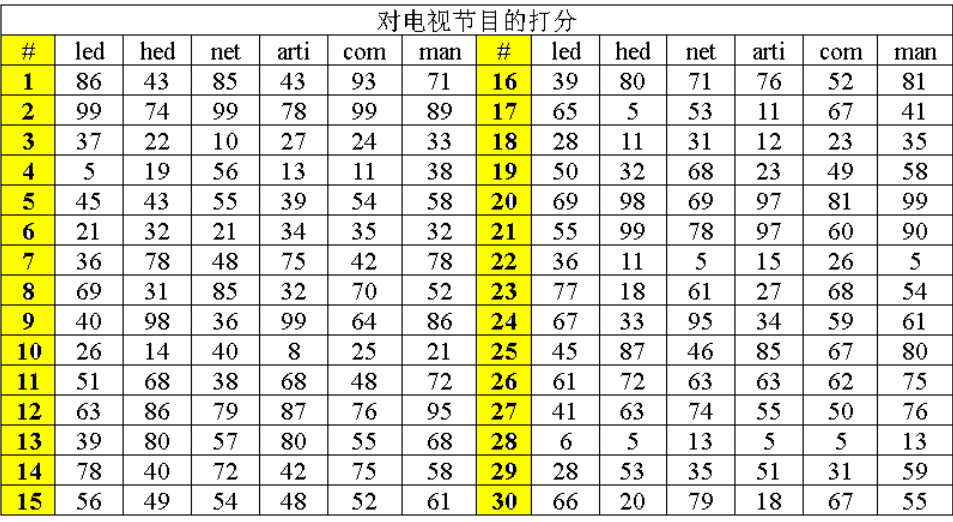### 1.1.1 发现问题

通过上表我们来探究观众和业内人士对一些电视节目的观点之间存在着什么关系，其中观众评分来自低学历（led）、高学历（hed）和网络（net）调查三种，他们形成第一组变量；而业内人士评分来自包括演员和导演在内的艺术家（art）、发行（com）与业内各部门主管（man）三种，形成第二组变量
我们直接对这些变量的相关进行两两分析，很难得到关于这两组变量（观众和业内人士）之间关系的一个清楚的印象

### 1.1.2 解决思路

因此，我们需要把多个变量与多个变量之间的相关化为两个具有代表性的变量之间的相关

### 1.1.3 选出代表

代表`:能较为综合、全面的衡量所在组的内在规律
一组变量最简单的综合形式就是该组变量的线性组合

# 二 典型相关分析

## 2.1 典型相关分析的定义

典型相关分析的基本思想和主成分分析十分相似
它按以下步骤进行分析：

1. 首先在每组变量中找出这些变量的线性组合，使得两组的线性组合之间具有很大的相关系数
2. 然后选取和最初挑选的这对线性组合不相关的线性组合，使其配对，并选取相关系数最大的一堆
3. 如此继续下去，直到两组变量之间的相关性被提取完毕为止
4. 被选出的线性组合配对称为典型变量，它们的相关系数被称为典型相关系数。典型相关系数度量了这两组变量之间联系的强度

## 2.2 典型相关分析的思路

假设两组变量分别为： X 1 = ( X 1 1 、 X 2 1 . . . X p 1 ) ， X 2 = ( X 1 2 、 X 2 2 . . . X q 2 ) X^{1}=(X_{1}^{1}、X_{2}^{1}...X_{p}^{1})，X^{2}=(X_{1}^{2}、X_{2}^{2}...X_{q}^{2})
分别在两组变量中选取若干有代表性的综合变量 U i 、 V i U_{i}、V_{i} ,
使得每一个综合变量是原变量的线性组合，即
U i = a 1 ( i ) X 1 ( 1 ) + a 2 ( i ) X 2 ( 1 ) + . . . + a p ( i ) X p ( 1 ) ≡ a ( i ′ ) X ( 1 ) V i = b 1 ( i ) X 1 ( 2 ) + b 2 ( i ) X 2 ( 2 ) + . . . + b q ( i ) X q ( 2 ) ≡ b ( i ′ ) X ( 2 ) U_{i} = a_{1}^{(i)}X_{1}^{(1)}+a_{2}^{(i)}X_{2}^{(1)}+...+a_{p}^{(i)}X_{p}^{(1)} \equiv \boldsymbol {a^{(i')}X^{(1)}} \\ V_{i} = b_{1}^{(i)}X_{1}^{(2)}+b_{2}^{(i)}X_{2}^{(2)}+...+b_{q}^{(i)}X_{q}^{(2)} \equiv \boldsymbol {b^{(i')}X^{(2)}} \\
注意：综合变量的组数是不确定的，如果第一组就能代表原样本数据大部分的信息，那么一组就足够了。假设第一组反应的信息不够，我们才需要找第二组了。
并且为了让第二组的信息更有效，需要保证两组信息不相关
不 相 关 ： c o v ( U 1 , U 2 ) = c o v ( V 1 , V 2 ) = 0 不相关：cov(U_{1},U_{2})=cov(V_{1},V_{2})=0
**第一组要满足的条件：**在 v a r ( U 1 ) = v a r ( V 1 ) = 1 var(U_{1})=var(V_{1})=1 满足的条件下，找到 a 1 a^{1} b 1 b^{1} 两组系数，使得 ρ ( U 1 , V 1 ) \rho(U_{1},V_{1}) 最大
为什么要固定这个条件呢？
因为相关系数与量纲无关： ρ ( U 1 , V 1 ) = ρ ( a U 1 , b V 1 ) \rho(U_{1},V_{1})=\rho(aU_{1},bV_{1})

## 2.3典型相关分析关键步骤1

1. 数据的分布有假设：两组数据服从联合正态分布
在实际分析应用中，总体的
协差阵
通常是未知的，往往需要从研究的总体中随机抽取一个样本，根据样本估计出总体的协差阵，并在此基础上进行典型相关分析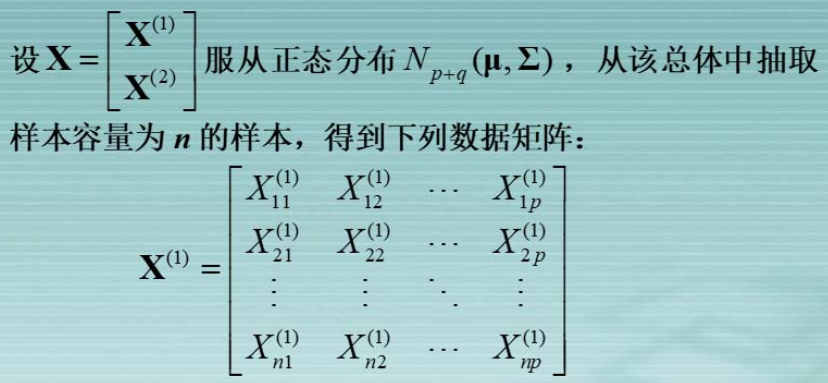2. 首先要对两组变量的相关性进行检验（构造似然比统计量），p值小于0.05（0.1）表示在95%（90%）的置信水平下拒绝原假设，即认为两组变量有关
在利用样本进行两组变量的典型相关分析时，应该就两组变量的相关性进行检验。这是因为，如果两个随机变量 X 1 、 X 2 X^{1}、X^{2} 互不相关，则两组变量协差阵 C o v ( X ( 1 ) , X ( 2 ) ) = 0 Cov(X^{(1)},X^{(2)})=0 .但是有可能得到的两组变量的样本协差阵不为0.
因此，在用样本数据进行典型相关分析的时候应该就两组变量的协差阵是否为0进行检验。即检验假设
H 0 : ∑ 12 = 0 , H 1 : ∑ 12 ≠ 0 H_{0}:\sum_{12}=0, \qquad H_{1}:\sum_{12}\neq 0
根据随机向量的检验理论可知，用于检验的似然比统计量为
Λ 0 = ∣ ∑ ^ ∣ ∣ ∑ ^ 11 ∣ ∣ ∑ ^ 22 ∣ = Π i = 1 r ( 1 − λ ^ 2 ) \Lambda_{0}=\frac{\vert \hat{\sum} \vert}{\vert \hat{\sum}_{11}\vert \vert\hat{\sum}_{22}\vert}=\mathop\Pi\limits_{i=1}^{r}(1-\hat{\lambda}^{2})
3. 确定典型相关变量的个数（直接看典型相关系数对应的P值即可）
如果总体典型相关系数 λ k = 0 \lambda_{k}=0 ,则相应的典型变量 U k , V k U_{k},V_{k} 之间无相关关系，因此对于分析 X ( 1 ) X^{(1)} X ( 2 ) X^{(2)} 的影响不起作用，这样的典型变量可以不予考虑，于是提出如何根据样本资料判断总体典型相关系数是否为零，以便确定应该取几个典型变量的问题。
巴特莱特（Bartlett）提出了一个根据样本数据检验总体典型相关系数 λ 1 、 λ 2 、 . . . 、 λ r \lambda_{1}、\lambda_{2}、...、\lambda_{r} 是否等于零的方法。检验假设为：
H 0 : λ k + 1 = λ k + 2 = . . . = λ r = 0 H 1 : λ k + 1 ≠ 0 用 于 检 验 的 似 然 比 统 计 量 为 : Λ k = Π i = k + 1 r ( 1 − λ i 2 ^ ) H_{0}:\lambda_{k+1}=\lambda_{k+2}=...=\lambda_{r}=0 \\ H_{1}:\lambda_{k+1}\neq 0 \\ 用于检验的似然比统计量为:\\ \Lambda_{k} = \mathop\Pi\limits_{i=k+1}^{r}(1-\hat{\lambda_{i}^{2}})
4. 利用标准化后的典型相关变量分析问题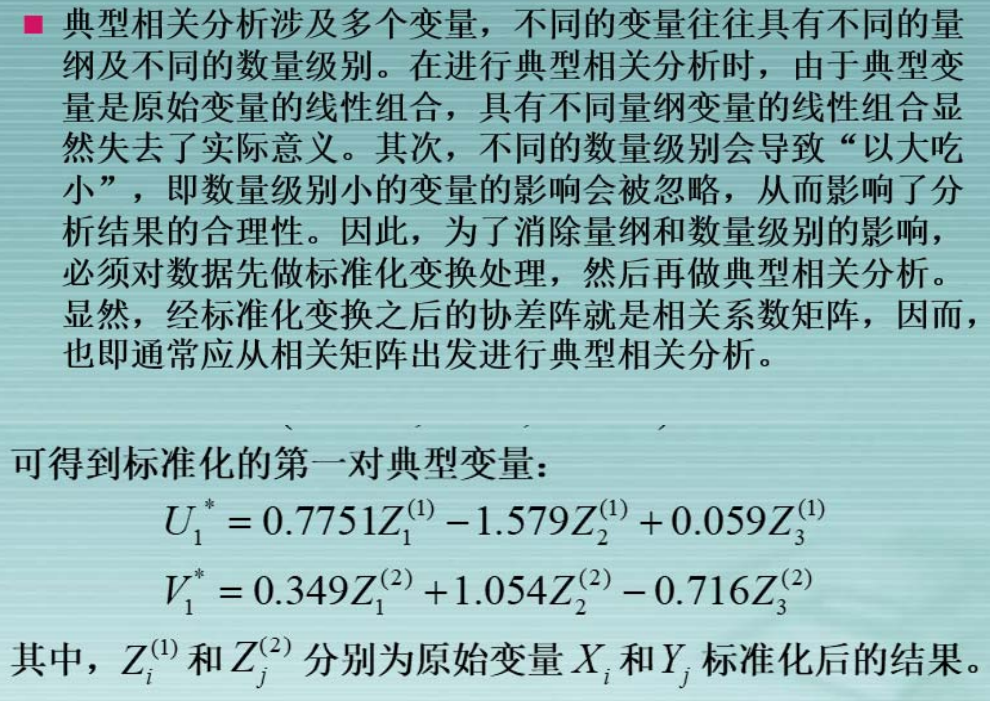5. 进行典型载荷分析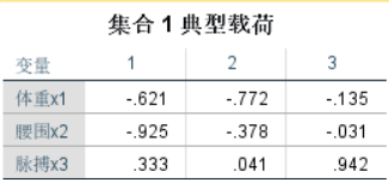以上结果说明了生理指标的第一典型变量与体重的相关系数为**-0.621**，与腰围的相关系数为**-0.925**，与脉搏的相关系数为0.333，这个从另一方面说明了生理指标的第一对典型变量与体重、腰围负相关，而与脉搏正相关。其中与腰围的相关性最强，因此第一对典型变量主要反映了体形的胖瘦
6. 计算前r个典型变量对样本总方差的贡献
由上图计算得
第 一 典 型 变 量 解 释 的 方 差 比 例 ： ( 0.62 1 2 + 0.92 5 2 + 0.33 3 2 ) / 3 = 0.451 第 二 典 型 变 量 解 释 的 方 差 比 例 ： ( 0.77 2 2 + 0.37 7 2 + 0.04 1 2 ) / 3 = 0.246 第 三 典 型 变 量 解 释 的 方 差 比 例 ： ( 0.13 5 2 + 0.03 1 2 + 0.94 2 2 ) / 3 = 0.302 第一典型变量解释的方差比例：\\ (0.621^2 + 0.925^2 + 0.333^2)/3 = 0.451\\ 第二典型变量解释的方差比例：\\ (0.772^2 + 0.377^2 + 0.041^2)/3 = 0.246\\ 第三典型变量解释的方差比例：\\ (0.135^2 + 0.031^2 + 0.942^2)/3 = 0.302\\

# 三 SPSS操作步骤

Spss至少需要24版本。低版本不能直接进行典型相关分析的操作，需要编程
第一步：导入数据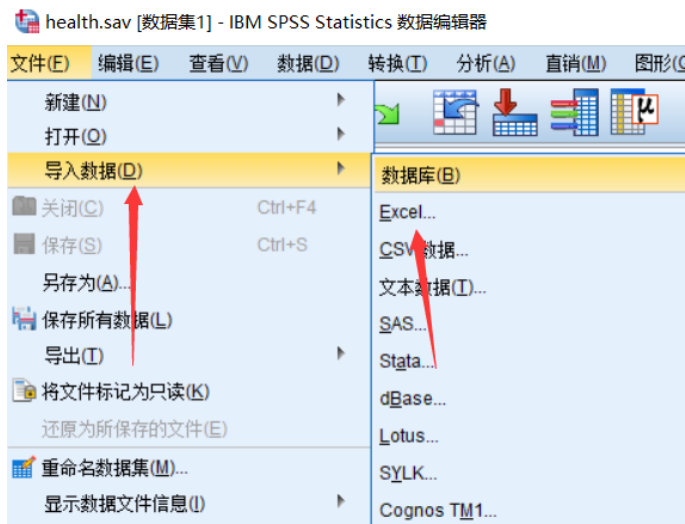第二步：检验数据的类型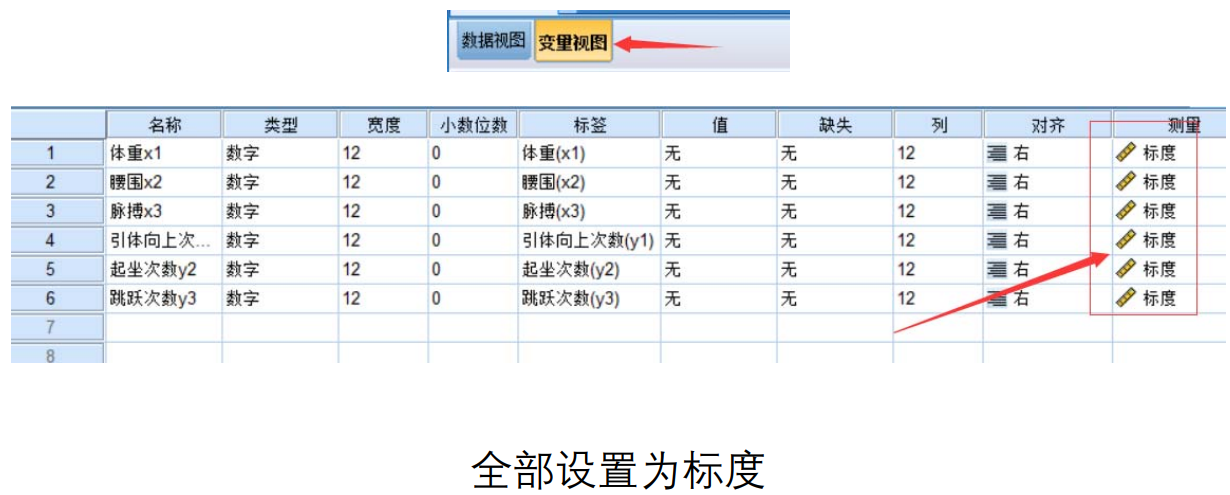第三步：点击菜单功能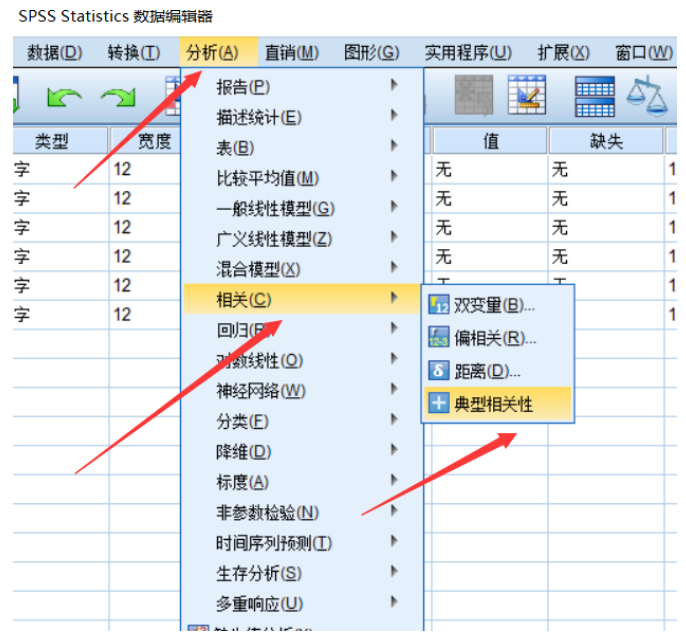第四步：将数据移动到对应的集合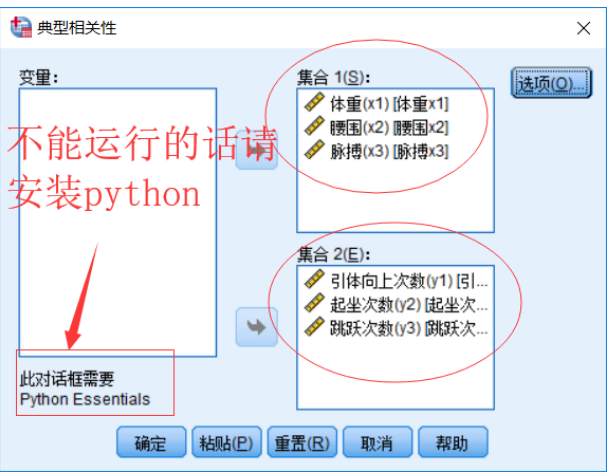第五步：导出分析结果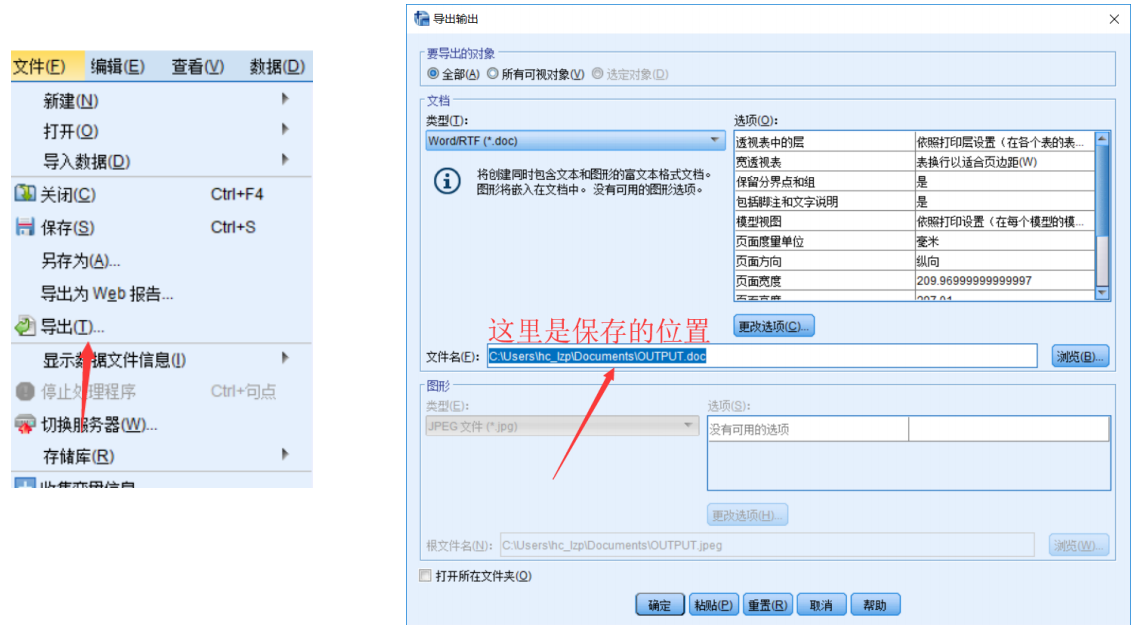第六步：对结果进行分析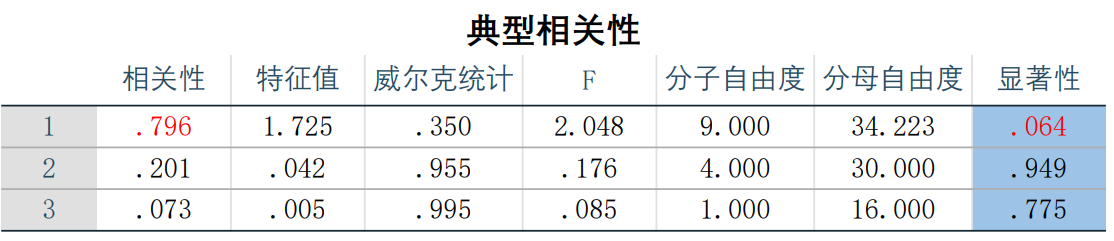这里表格最后一列表示检验统计量所对应的p值，要通过它确定典型相关系数的个数，过关的只有第一对，将它对应的集合的相关系数表示为：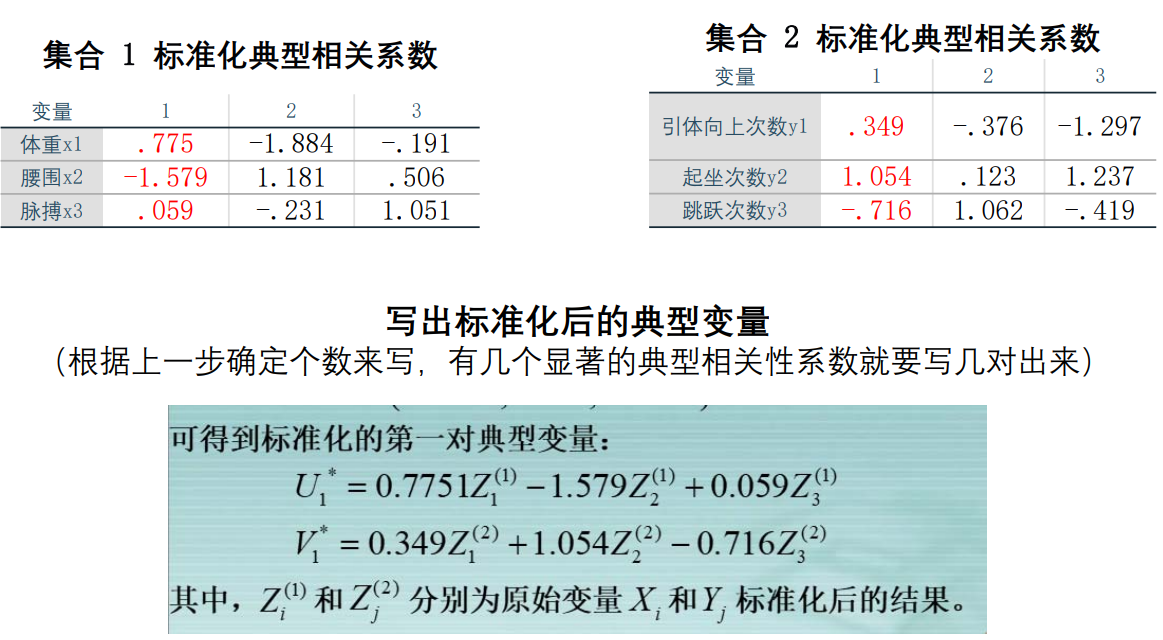典型变量每个分量前面的系数代表重要程度，可以结合典型相关系数进行分析
之后选择性的分析典型载荷和方差解释程度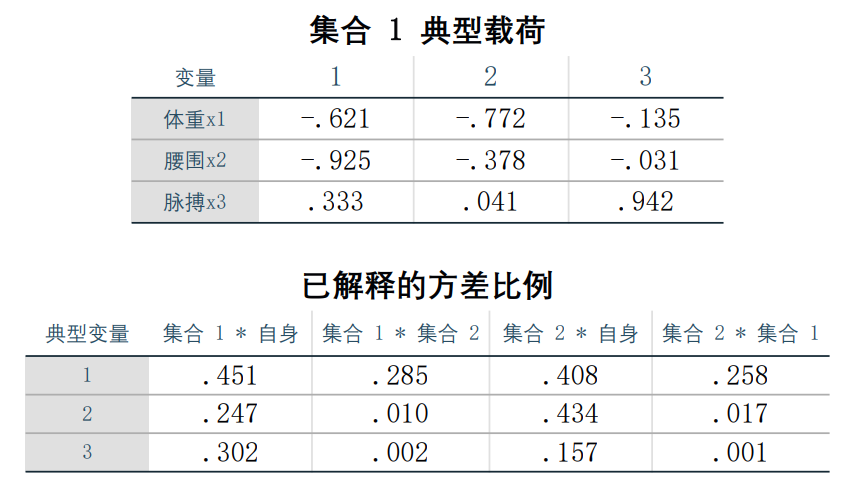1. 参考资料：厦门大学—多元统计分析第九章典型相关分析.ppt ↩︎

展开全文• 文章目录一、引述1.概念2.示例说明 一、引述 ...典型相关分析用于研究两组变量（每组变量中都可能有多个指标）之间相关关系的一种多元统计方法。它能够揭示出两组变量之间的内在联系。 2.示例说明 ...
• 一、典型相关分析VS皮尔逊相关系数/斯皮尔曼相关系数 典型相关分析（Canonical Correlation analysis） 研究两组变量（每组变量中都可能有多个指标） 之间相关关系的一种多元统计方法。它能够揭示出两组变量之间的...
• 典型相关分析一.应用二.思想三.过程四.关键步骤五.spss操作第一步：导入数据第二步：检验数据的类型第三步：点击菜单功能第四步：将数据移动到对应的集合第五步：导出分析结果第六步：改名六.结果分析第一步：数据的...python 算法 matlab
• ## 典型相关分析原理（CCA）

万次阅读 多人点赞 2020-01-21 12:29:17
CCA典型相关分析 （canonical correlation analysis）利用综合变量对之间的相关关系来反映两组指标之间的整体相关性的多元统计分析方法。它的基本原理是：为了从总体上把握两组指标之间的相关关系，分别在两组变量中...
• 今天，小编将从数据分析的最基本概念和数据分析基本步骤两个方面入手，谈谈数据分析。 一、数据分析的概念 数据分析就是运用恰当的分析方法，分析所收集的海量资料，并运用高效的分析工具将之归类、归纳，从中提炼...大数据
• 4对典型相关变量中只有第1对典型相关变量的相关系数的达到显著水平，所以本例中只用第1对典型相关变量的数据来解释X变量和Y变量之间的关系。2.X的5个观察变量中，观察变量x5对第1典型变量U1的关系最紧...
• 1.典型相关分析：两组变量之间的相关问题 cancor() cancor(x, y, xcenter = TRUE, ycenter = TRUE) x，y为两组变量的数据矩阵；xcenter和ycenter是逻辑值，表示是否中心化，实际中一般采用默认值TRUE 注意...
• 记录一下R语言学习过程，对于R的基础就是基本数据类型（向量，矩阵，数据框，字符串等等），库的调用以及函数自定义，还需要多加学习！ 进入主题，今天主题是相关性分析： 以下为代码： y<-c(170,175,180) //定义...
• 典型相关分析（Canonical Correlation Analysis）是研究两组变量之间相关关系的一种多元统计方法，它能够揭示出两组变量之间的内在联系。         在一元统计分析中，用相关系数来...
• 来看变量之间是否存在相关关系，以及可以通过DW检验，来看变量之间是否存在自相关关系。 第四步：变量转换：包含方差稳定转换或BOX-COX转换 第五步：模型检验：残差分析 第六步：模型检验：极端...
• 8.3 因子载荷矩阵的估计方法(一)主成分分析法回顾一下主成分法估计因子载荷矩阵的步骤：求出原变量协方差阵(或相关阵)的前 m 个特征根(考虑累积贡献率)，后面的特征根忽略掉因子载荷矩阵的每一列为前 m 个特征根乘上...
• 典型相关分析基本思想 Canonical Correlation Analysis   CCA典型相关分析 （canonical correlation analysis）利用综合变量对之间的相关关系来反映两组指标之间的整体相关性的多元统计分析方法。它的基本原理...CCA
• 相关分析，两个变量之间密切程度的一种常见统计分析方法，能够简单有效说明两变量间存在什么关系，这些关系的常见描述语句有：线性相关、正相关、负相关等。 【数据集说明】 某公司员工的基本情况，数据集含3...
• 结构化分析具体步骤 1.建立当前系统的“具体模型”：分析系统的“具体模型”，就是现实环境的忠实写照，这样的表达与当前系统完全对应，因此用户容易理解。 2.抽象出当前系统的逻辑模型：分析系统的"具体模型“，...http
• ​​​​​​​大数据业务分析基本步骤 ​​​​​​​明确分析目的和思路 ​​​​​​​数据收集flumesqoopkettle ​​​​​​​数据处理-ETLMapReduceSpark ​​​​​​​数据分析HiveSQLSparkSQL 数据...
• ## 典型相关分析（CCA）

万次阅读 多人点赞 2014-09-14 22:12:17
典型相关分析基本思想 Canonical Correlation Analysis CCA典型相关分析 （canonical correlation analysis）利用综合变量对之间的相关关系来反映两组指标之间的整体相关性的多元统计分析方法。它的基本原理...
• ## 时间序列相关算法与分析步骤

万次阅读 多人点赞 2018-04-06 11:13:02
首先，从时间的角度可以把一个序列基本分为3类：1.纯随机序列（白噪声序列），这时候可以停止分析，因为就像预测下一次硬币哪一面朝上一样毫无规律。2.平稳非白噪声序列，它们的均值和方差是常数，对于这类序列，有...时间序列 自回归 移动平均 ARMA
• ## 典型相关分析相关资料

万次阅读 多人点赞 2012-05-20 17:29:14
典型相关分析基本思想 Canonical Correlation Analysis   CCA典型相关分析 （canonical correlation analysis）利用综合变量对之间的相关关系来反映两组指标之间的整体相关性的多元统计分析方法。它的基本原理...variables pair
• 文章目录1.序2.动态规划的基本概念[^1]3.动态规划算法的基本思想[^2]4....这篇文章主要介绍动态规划算法的基本思想、使用动态规划算法求解问题的基本步骤、动态规划算法的两个基本要素以及一些经典的动态规划问题。...动态规划
• 典型相关分析基本思想 Canonical Correlation Analysis CCA典型相关分析 （canonical correlation analysis）利用综合变量对之间的相关关系来反映两组指标之间的整体相关性的多元统计分析方法。它的基本原理是...
• 相关性分析研究现象之间是否存在某种依存关系，对具体有依存关系的现象探讨相关方向及相关程度。 相关分析是一种简单易行的测量定量数据之间的关系情况的分析方法。可以分析包括变量间的关系情况以及关系强弱程度...大数据 变量
• 数据分析学习总结笔记06：T检验1 单样本T检验1.1 单样本T检验概念1.2 单样本T检验步骤2 独立样本T检验2.1 独立样本T检验概念2.2 独立样本T检验步骤 1 单样本T检验 1.1 单样本T检验概念 目的：利用来自某总体的样本...数据挖掘大数据 大数据学习 大数据入门...